# Rolling hash, Rabin Karp, palindromes, rsync and othersCosmin Negruseri
30 octombrie 2012

Rolling hash is a neat idea found in the Rabin-Karp string matching algorithm which is easy to grasp and useful in quite a few different contexts.

As before, the most interesting part of the article is the problem section. Discuss them in the comment section, but first let's go through a few applications.

## The Rabin-Karp algorithm

Given a string P of length n and a string S of length m find out all the occurrences of P within S

The algorithm works by building a fingerprint for each substring of S of length n. It checks if any of them match the fingerprint of P. Implementing this directly leads to a O(n*m) solution which isn't faster than the naive matching algorithm (in fact it may be slower).

The insight is that you can efficiently compute the hash value of a substring using the hash value of previous substring if you use the right hash function. A polynomial with the string characters as coefficients works well. Let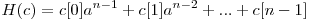be the hash function for the string c.
All the operations are done modulo a prime number so that we don’t have to deal with large integers.

Let’s see what happens when we go from H(S[i..i+n-1]) to H(S[i+1..i+n]):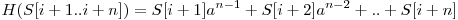Adding and substracting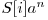we get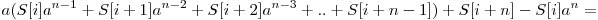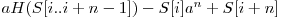Deleting a character and appending another one corresponds to adding a number and subtracting a number in our hashing algorithm. The complexity of the algorithm is O(n).

If S and P are strings of digits and a is 10, our problem maps to finding numbers in a string of digits.
Let's go through an example.

``````Let P = 53424, S = 3249753424234837 and a = 10
3249753424234837
32497
24975 = 10 * 32497 - 3 * 10000 + 5
49753 = 10 * 24975 - 2 * 10000 + 3
97534 = 10 * 49753 - 4 * 10000 + 4
...
53424 // match
...``````

Here's some code:

``````an = 1
rolling_hash = 0
for i in range(0, n):
rolling_hash = (rolling_hash * a + S[i]) % MOD
an = (an * a) % MOD
if rolling_hash == hash_p:
print "match"
for i in range(1, m - n + 1):
rolling_hash = (rolling_hash * a + S[i + n - 1] - an * S[i - 1]) % MOD
if rolling_hash == hash_p:
print "match"``````

## Longest common substring

Given two strings A and B, compute their longest common substring.

Let's solve a simpler problem: Given two strings A and B, and a number X find if they have a common sequence of length X. We use the rolling hash technique to find the hash codes for all X length substrings of A and B. Then we check if there is any common hash code between the two sets, this means A and B share a sequence of length X. We then use binary search to find the largest X.
The complexity of this algorithm is O(n log n).

## rsync

The linux utility is used to keep two copies of the same file in sync by copying around deltas. Instead of replicating the whole changed file, rsync segments the file into fixed size chunks, computes a rolling hash for each chunk. On the client side computes rolling hashes for all chunks of the target file including overlapping ones. Then it checks which chunks are already there, which chunks need to be deleted and which chunks need to be copied over. This improves the transfer speed as you only copy over deltas and some chunk fingerprints. Here's a better description from wikipedia.

## Largest palindrome

Given a string of length n, find out its largest palindromic substring.

The naive solution is O(n3). For every possible substring it tests in linear time if it's a palindrome.

A smarter solution tries every position in the original string as the center of a palindrome and extends to the left and to the right as long as the corresponding characters match. This solution takes O(n2) time.

There's a O(n log n) solution that involves rolling hash. Given X we can test if there is any substring S' of S of length 2X such that H(S') == H(reverse(S')). The identity means that S contains a palindromic sequence of length at least 2X. What is left is to find out the maximum such X. We can use binary search to do that efficiently. The overall complexity is O(n log n).

## Substrings of variable length

The polynomial function can be used to compute hashes for substrings of varying length

Let’s choose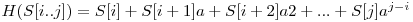Then we compute the array h which contains cummulative hashes: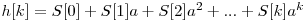. It’s easy to see that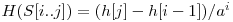After spending O(n) time on preprocessing h, we have an efficient way to compute the hash code for any substring of S.

The division operation modulo a prime needs some number theory insight. You can do it using Fermat's little theorem or using the Extended Euclidean Algorithm. If you need to process a lot of sub strings it's useful to pre process all the values for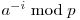.

## Caveats

Hashing collisions are a problem. One way to deal with them is to check for a match when two fingerprints are equal, but this makes the solution inefficient if there are lots of matches. Another way is to use more hashing functions to decrease the collision probability.

## Alternatives

Suffix trees or suffix arrays are very good tools to tackle string matching problems. I prefer hashing as the code is usually simpler.